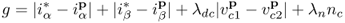# 5.4 Predictive Current Control Method

The predictive control scheme for the NPC inverter is shown in Figure 5.7. The future values of the load currents and voltages in the capacitors are predicted for the 27 switching states generated by the inverter, by means of (5.17), (5.11), and (5.12). For this purpose, it is necessary to measure the present load currents and voltages in the capacitors. After obtaining the predictions, a cost function g is evaluated for each switching state. The switching state that minimizes the cost function is selected and applied during the next sampling period.

Figure 5.7 Predictive current control method for the NPC inverter (Vargas et al., 2007 © IEEE)The control requirements for the NPC inverter are:

5.19The first two terms are the load current errors in orthogonal coordinates, whereandare ...